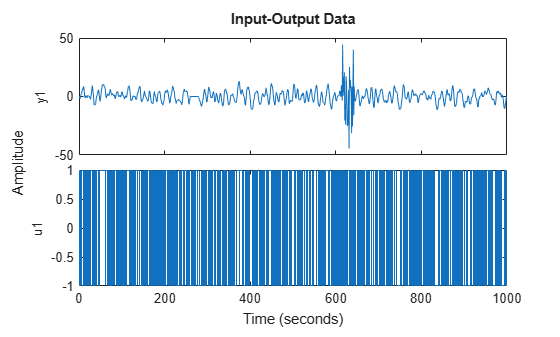# merge (iddata)

Merge data sets into iddata object

## Syntax

```dat = merge(dat1,dat2,....,datN)
```

## Description

`dat` collects the data sets in ```dat1, ...,datN``` into one `iddata` object, with several experiments. The number of experiments in `dat` will be the sum of the number of experiments in `datk`. For the merging to be allowed, a number of conditions must be satisfied:

• All of `datk` must have the same number of input channels, and the `InputNames` must be the same.

• All of `datk` must have the same number of output channels, and the `OutputNames` must be the same. If some input or output channel is lacking in one experiment, it can be replaced by a vector of `NaN`s to conform with these rules.

• If the `ExperimentNames` of `datk` have been specified as something other than the default `'Exp1'`, `'Exp2'`, etc., they must all be unique. If default names overlap, they are modified so that `dat` will have a list of unique `ExperimentNames`.

The sampling intervals, the number of observations, and the input properties (`Period`, `InterSample`) might be different in the different experiments.

You can retrieve the individual experiments by using the command `getexp`. You can also retrieve them by subreferencing with a fourth index.

```dat1 = dat(:,:,:,ExperimentNumber) ```

or

```dat1 = dat(:,:,:,ExperimentName) ```

Storing multiple experiments as one `iddata` object can be very useful for handling experimental data that has been collected on different occasions, or when a data set has been split up to remove “bad” portions of the data. All the toolbox routines accept multiple-experiment data.

## Examples

collapse all

Remove bad portions of data to estimate models without the bad data destroying the estimate.

```load iddemo8; plot(dat);```Bad portions of data are detected around sample 250 to 280 and between samples 600 to 650. Cut out these bad portions to form a multiple-experiment data set and merge the data.

`dat = merge(dat(1:250),dat(281:600),dat(651:1000));`

You can use the first two experiments to estimate a model and the third experiment to validate the model.

```dat_est = getexp(dat,[1,2]); m = ssest(dat_est,2); dat_val = getexp(dat,3);```